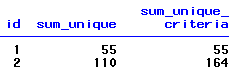# SAS SQL : Use Distinct in CASE WHEN

This tutorial explains how to ignore duplicates while specifying conditions / criteria in SQL queries. You must have used DISTINCT keyword to remove duplicates. It is frequently used with COUNT function to calculate number of unique cases.

Example 1 :

Suppose you have three variables, say, 'id', 'x' and 'y'. You need to calculate number of distinct "y" values when x is less than 30. See the snapshot of data below -Dummy Dataset : CASE WHEN

Let's create dataset in SAS
data temp;
input id x y ;
cards;
1 25 30
1 28 30
1 40 25
2 23 54
2 34 54
2 35 56
;
run;

SAS : PROC SQL
proc sql;
select count(distinct y) as unique_y,
count(distinct case when x < 30 then y else . end) as unique_criteria
from temp;
quit;Output
Explanation :
1. The above program computes number of distinct values in variable 'y' when values of variable "x" is less than 30.
2. The keyword DISTINCT is used to remove or ignore duplicate records.
3. In the dataset, there are in total 3 cases in variable 'y' when x < 30. Whereas distinct number of cases in variable 'y' is equal to 2.

Example 2 :

Suppose you are asked to group values by ID and then calculate sum of distinct values of y when x < 30. If condition is not met, then sum of all values of y.
proc sql;
select id, sum(distinct y) as sum_unique,
coalesce(sum(distinct case when x < 30 then y end),0) +
coalesce(sum(case when x >= 30 then y end),0) as sum_unique_criteria

from temp
group by 1;
quit;Output : Example 2

Explanation :
1. Since the DISTINCT keyword works on a complete record, we need to write conditions "x <30" and "x>=30" separately in CASE WHEN.
2. The COALESCE function tells SAS to replace missing values with 0 and then sum the returned values of both the conditions. If we don't use COALESCE, it would return missing when any of the two values which we want to add contains missing/null.
Example 3 :

Suppose you are asked to group data by variable 'ID' and then calculate maximum value of variable 'Y' when x is less than 30. Otherwise take all the values. At last, sum the returned values of both the conditions.Example 3 : Case When
data temp;
input id x y ;
cards;
1 25 30
1 28 27
1 40 25
2 23 54
2 29 55
2 34 56
;
run;
proc sql;
select id,
coalesce(max(case when x < 30 then y end),0) +
coalesce(sum(case when x >= 30 then y end),0) as sum_unique_criteria
from temp
group by 1;
quit;
Example 4 :

Suppose you need to pick the maximum value in variable Y when duplicates in variable "X" and then group data by variable "ID" and compute number of cases where Y=1.Example 4 : CASE WHEN with DISTINCT
data temp;
input id x y ;
cards;
1 1 1
1 1 0
1 2 1
2 3 0
2 4 1
2 4 1
;
run;
proc sql;
select a.id,
count(distinct case when y > 0 then max_y else . end) as count_distinct
from temp a left join (select x, max(ranuni(123) * y) as max_y from temp group by 1) b
on a.x = b.x
group by 1;
quit;

How it works :
1. When X = 1, it picks the maximum value of variable Y i.e. 1 and sets Y =1. Then it groups data by variable "ID", it checks the number of cases in which Y is equal to one after removing duplicates in X=1 cases. So it returns 2.
2. The RANUNI() function is used to generate random numbers between 0 and 1 without replacement.The number 123 that is enclosed in the ranuni function is called seed which produces the same random numbers when it is run next time.
3. In this case, the RANUNI() function makes Y as unique identifier so that we can later count these unique cases.Share
Related PostsDeepanshu founded ListenData with a simple objective - Make analytics easy to understand and follow. He has over 10 years of experience in data science. During his tenure, he has worked with global clients in various domains like Banking, Insurance, Private Equity, Telecom and Human Resource.

10 Responses to "SAS SQL : Use Distinct in CASE WHEN"
1.Simply superb article thank you

2.Hi - I don't understand the last SQL query - can you further explain?

3.Superb article

4.I have looked all over for the process to just "ignore" duplicate rows in Spotfire. Everything I find it about writing long expressions and inseting new columns to remove duplicate rows. this is the simple and easy button solution Fantastic!

5.data ss;
input id;
datalines;
1
2
3
4
5
-1
-2
-3
-4
-5
;
run;

i want sum of positive value in one variable and negative value in another variable

1.data sum1 minus1;
set ss;
if id >=0 then output sum1;
else output minus1;
run;

6.data want;
set ss;
retain positive negative 0;
if id>0 then positive+a;
else negative+a;
run;

1.can we use firstobs and obs concept

7.This comment has been removed by the author.

8.Good post, if anyone stumbles upon this and want to know basics of SQL case then you can check examples in below link

https://qawithexperts.com/article/sql/understanding-sql-server-switch-case-with-example/147

Thanks

Next → ← Prev